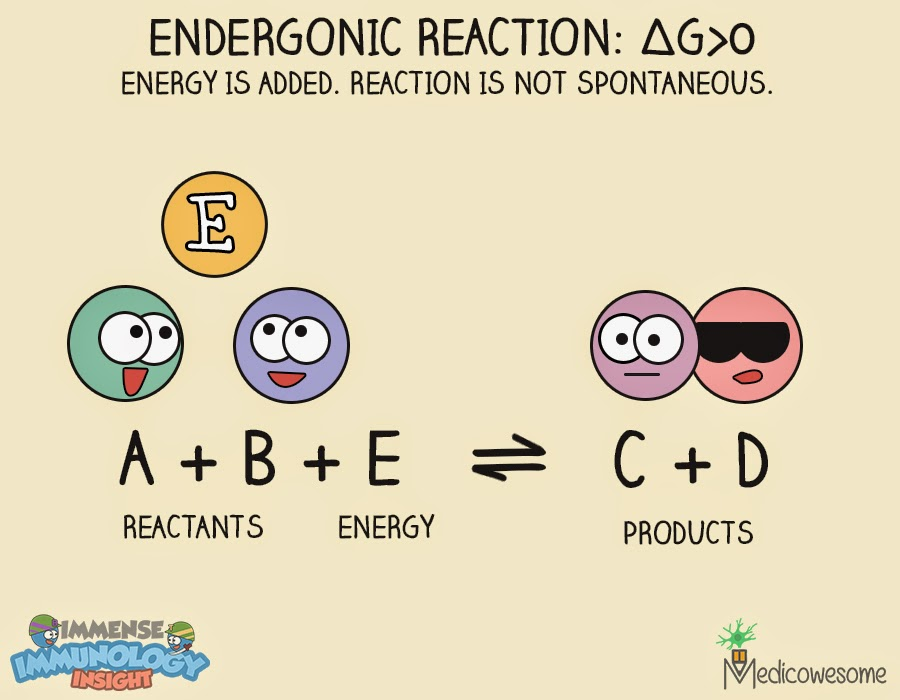## Thursday, April 23, 2015

### Entropy, Enthalpy and Gibbs free energy – The monsters of bioenergetics.

The first law of thermodynamics is very famous. It says, "Energy can neither be created nor be destroyed but is converted from one form to another."

So simple.

But can you explain the second law of thermodynamics? A bit puzzled, aren't you?

It's the very reason I call it the monsters of bioenergetics. Let's convert these monsters into cute little pixies :)

The second law of thermodynamics says, "The entropy of the universe goes on increasing over time."

What is entropy?
Entropy is the degree of randomness.

A solid has closely placed molecules. Hence, the randomness in molecules is less. On the on the other hand, in liquids, the distance between the molecules is more. Hence, they have more randomness and more entropy value.

Melting of ice is a good example which illustrates the second law of thermodynamics. When the ice melts, solid gets converted into it's liquid form. The distance between the molecules increases from solid to liquid and thus, the entropy increases!

Here's an interesting fact: The human body consumes carbohydrates, breaks it down and stores its energy as ATP, which is a high energy molecule. One would argue that storage of such high energy molecule is against the second law, as entropy of the body is not increasing in this reaction. The entropy increases, but in this case, the entropy of the universe increases because we release carbon dioxide into the surrounding!

Since we are on this topic, let's address two more terms - Gibbs free energy and enthalpy!

Gibbs free energy

It is the Gibbs free energy which determines whether the reaction will proceed spontaneously to equilibrium without any input from surrounding.

In a reaction, if reactants are unstable (Having more energy) and the products are stable (Having less energy), then the reaction tends to move forward spontaneously without any input from surrounding.

On the other hand, if reactant is more stable than products then for this reaction to happen there has to some input of energy from surrounding.

Hence, if products have less Gibbs free energy than the reactants (i.e. change in Gibbs free energy is negative) then the reaction is spontaneous/exergonic irrespective of whether it is exothermic or endothermic.Exergonic reaction - Thermodynamics
If products have more Gibbs free energy than the reactants (i.e. change in Gibbs free energy is positive) then the reaction is non-spontaneous/endergonic.Endergonic reaction - Thermodynamics
Enthalpy

Enthalpy (H) is a sum of useful energy and non-useful energy. The non-useful part is the Entropy (S) and the useful part is the Gibbs free energy (G).

ΔH = ΔG + TΔS

To summarize all the three terms:
Entropy: Degree of randomness (Non-useful energy)
Gibbs free energy: Energy available to do work (Useful energy)
Enthalpy: Sum of Entropy and Gibbs free energy!

Illustrated by Immense Immunology Insight
Related post: How to remember the sign and direction of Gibbs free energy change

1.Very well written Komal! Quite interesting is the way u explained it, simplifying and making it easy to understand! :)

1.2.Very well explained. U make me proud!!

3.:D
thank u mam! :)
its only bcoz of U!

This is express yourself space. Where you type create something beautiful! <3
Wondering what do I write? Well...
Tell us something you know better. You are a brilliant mind. Yes, you are! ^__^